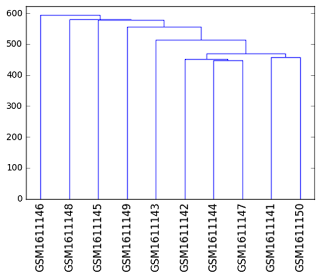# Categorical labels on numerical axis?

Is it possible to put text labels on a numerical axis? I’m trying to plot a dendogram (matplot lib example below), and would like the axis labels to be categorical, however I still need numerical x-coordinates for plotting. I’ve been considering adding a categorical axis, or trying the FuncTickFormatter, but have had trouble finding relevant examples.Thanks for any help/ suggestions,

–Isaac

Figured it out from the v0.12.3 release notes.

import numpy as np

from scipy.cluster import hierarchy

from scipy.spatial import distance

from bokeh.plotting import figure, show, output_file

from bokeh.models import ColumnDataSource, FuncTickFormatter, FixedTicker

output_file(“test.html”)

data = np.random.rand(5, 300)

labels = list(“abcde”)

clusts = hierarchy.average(data)

dend = hierarchy.dendrogram(clusts, no_plot=True)

plot_ds = ColumnDataSource({“x”: dend[“icoord”],

“y”: dend[“dcoord”]})

axis_ds = ColumnDataSource({“label”: [labels[i] for i in dend[“leaves”]],

“position”: list( range(5, len(labels)*10+5, 10))})

p = figure(height=400, width=600)

p.multi_line(“x”, “y”, source=plot_ds)

p.xaxis.ticker = FixedTicker(ticks=axis_ds.data[“position”])

p.xaxis.formatter = FuncTickFormatter.from_coffeescript(

``````args=dict(axis_dsource=axis_ds),

code="""
``````

axis_data = axis_dsource.get(“data”)

pos = axis_data[“position”]

idx = pos.indexOf(tick) if tick in pos

axis_data[“label”][idx]

``````""")
``````

show§

···

On Thursday, October 13, 2016 at 2:01:25 PM UTC+11, [email protected] wrote:

Is it possible to put text labels on a numerical axis? I’m trying to plot a dendogram (matplot lib example below), and would like the axis labels to be categorical, however I still need numerical x-coordinates for plotting. I’ve been considering adding a categorical axis, or trying the FuncTickFormatter, but have had trouble finding relevant examples.Thanks for any help/ suggestions,

–Isaac

Thanks so much for
sharing your solution. It’s much appreciated.

···

On 10/19/16 3:59 AM, wrote:

[email protected]

Figured it out from the v0.12.3 release notes.

import numpy as np

from scipy.cluster import hierarchy

from scipy.spatial import distance

from bokeh.plotting import figure, show, output_file

``````        from bokeh.models import ColumnDataSource,
``````

FuncTickFormatter, FixedTicker

output_file(“test.html”)

data = np.random.rand(5, 300)

labels = list(“abcde”)

clusts = hierarchy.average(data)

dend = hierarchy.dendrogram(clusts, no_plot=True)

plot_ds = ColumnDataSource({“x”: dend[“icoord”],

“y”: dend[“dcoord”]})

``````        axis_ds = ColumnDataSource({"label": [labels[i] for i in
``````

dend[“leaves”]],

``````        "position": list( range(5,
``````

len(labels)*10+5, 10))})

p = figure(height=400, width=600)

p.multi_line(“x”, “y”, source=plot_ds)

``````        p.xaxis.ticker =
``````

FixedTicker(ticks=axis_ds.data[“position”])

p.xaxis.formatter = FuncTickFormatter.from_coffeescript(

args=dict(axis_dsource=axis_ds),

code="""

axis_data = axis_dsource.get(“data”)

pos = axis_data[“position”]

idx = pos.indexOf(tick) if tick in pos

axis_data[“label”][idx]

“”")

show§

``````         On Thursday, October 13, 2016 at 2:01:25 PM UTC+11,
``````

wrote:

``````  You received this message because you are subscribed to the Google
``````

Groups “Bokeh Discussion - Public” group.

``````  To unsubscribe from this group and stop receiving emails from it,
``````

send an email to [email protected].

``````  To post to this group, send email to [email protected]

``````

Sarah Bird
Developer, Bokeh

``````    [
![Continuum Analytics](http://docs.continuum.io/_static/img/ContinuumWordmark.png)
](http://continuum.io)
``````

[email protected]

``````            Is it possible to put text labels on a
``````

numerical axis? I’m trying to plot a dendogram (matplot
lib example below), and would like the axis labels to be
categorical, however I still need numerical
x-coordinates for plotting. I’ve been considering adding
a categorical axis, or trying the FuncTickFormatter, but
have had trouble finding relevant examples.Thanks for any help/ suggestions,

–Isaac